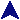Array AssignmentsCode Examples```use strict;

#
# This script demonstrates some of the properties of
# array assignments.
#
my @a = ("Small", "Medium", "Large");
print `@a = ` . "@a\n";

my (\$smith, \$jones, \$johnson) = @a;
print "\$smith \$jones \$johnson\n";

my (\$here, \$there) = @a;
print "\$here \$there\n";

my (\$this) = @a;
print "\$this\n";

my \$this = @a;
print "\$this\n";

my (\$p, \$q, \$r, \$s, \$t) = ("p", "q", "r", "s", "t");
print "[\$p] [\$q] [\$r] [\$s] [\$t]\n";
(\$p, \$q, \$r, \$s, \$t) = @a;
print "[\$p] [\$q] [\$r] [\$s] [\$t]\n";

# A cute way to swap variables.
my \$aa = "Fred";
my \$bb = "Barney";
(\$aa, \$bb) = (\$bb, \$aa);
print "\$aa \$bb\n";
```